UP TO 15 % DISCOUNT

Get Your Assignment Completed At Lower Prices

Plagiarism Free Solutions
100% Original Work
24*7 Online Assistance
Native PhD Experts
Hire a Writer Now
PC1201 Fundamental of Physics Assignment, NUS, Singapore: A car starts from 0 10 mx at 0 0 st and moves with the velocity graph shown in the figure below.
 University National University Singapore (NUS) Subject PC1201 Fundamental of Physics Assignment
Posted on: 30th Oct 2023

# PC1201 Fundamental of Physics Assignment, NUS, Singapore: A car starts from 0 10 mx at 0 0 st and moves with the velocity graph shown in the figure below.

1. A car starts from 0 10 mx  at 0 0 st  and moves with the velocity graph shown in the figure below.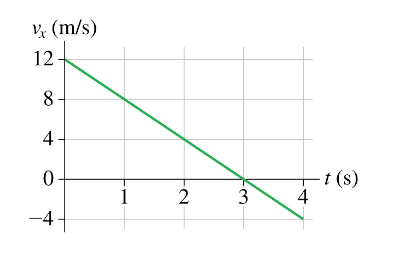(i) What is the car’s position at t = 2 s, 3 s, and 4 s?
(ii) Does this car ever change direction? If so, at what time?

2. Squid uses jet propulsion for rapid escapes. A squid pulls the water into its body and then rapidly ejects the water backward to propel itself forward. By ejecting 0.15 kg of water, a 1.5 kg squid (not including water mass) can accelerate at 20 m/s2.
(i) What is the magnitude of the thrust force on the squid?
(ii) What is the magnitude of the thrust force on the ejected water?
(iii) What acceleration is experienced by the ejected water?

3. Assuming no wind and we treat the air resistance to be negligible, the flight time of the symmetrical trajectory in the following figure is t.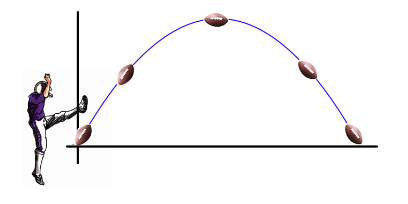Later, if a horizontal wind parallel to the ground is blowing, from right to left as in this figure, directly toward the player who kicks the ball, how would you expect the flight time of the ball to be affected if the ball does not spin while it is on the trajectory, if at all? Explain.

4. A block of mass m is released onto a vertical spring with spring constant k, where the lower end of the spring is fixed on a table. As the block falls, it will compress the spring until it momentarily comes to rest. Calculate the amount of compression in the spring if the block is released from a height h above the higher end of the spring as shown in the following figure. For the purposes of this calculation, we can treat the block as a point mass, and we will assume that the mass of the spring is negligible.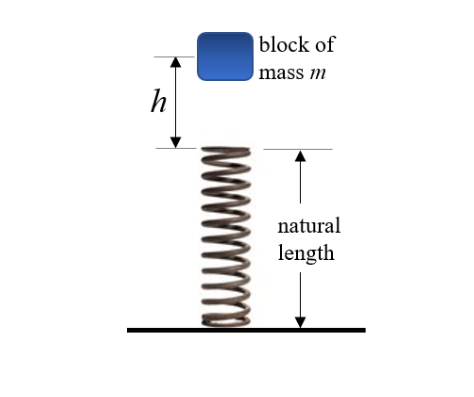##### Hire a Professional Essay & Assignment Writer for completing your Academic Assessments

5. An escalator is 60 m long and lifts passengers through a vertical height of 30 m as shown in the figure.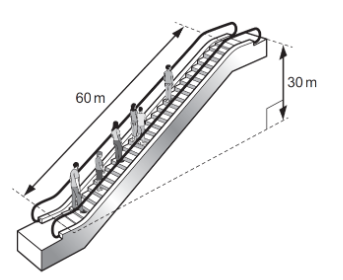To drive the escalator against the forces of friction when there are no passengers requires a power of 2.0 kW. The escalator is used by passengers of an average mass of 60 kg, and the power to overcome friction remains constant. How much power is required to drive the escalator when it is carrying 20 passengers and traveling at 0.75 m/s along the slope?

6. A spring with a spring constant of 2600 N/m is compressed 0.10 m from its unstretched position. The spring is released, propelling a 3.0-kg block along a horizontal, frictionless surface. This block then collides with a stationary 1.0-kg block. The blocks remain joined and move together as shown in the following figure.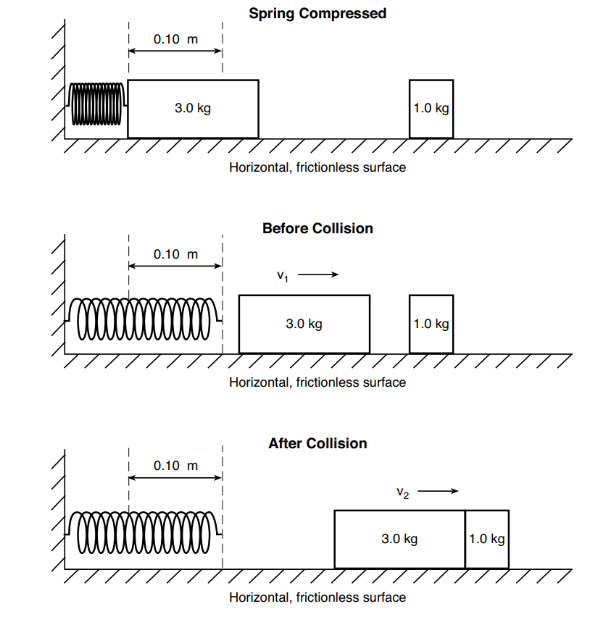(i) Determine the total amount of elastic potential energy stored in the spring when the spring is compressed 0.10 m.
(ii) Assuming all of the spring’s energy is transferred to the 3.0-kg block, calculate the speed v 1 of the 3.0-kg block immediately after it has left the spring.
(iii) Calculate the speed v 2 of the two blocks after the collision.
(iv) Qualitatively, explain why the collision is inelastic.

7. The ultrasonic transducer used in a medical ultrasound imaging device is a very thin disk (m = 0.10 g) driven back and forth in SHM at 1.0 MHz by an electromagnetic coil.
(i) The maximum restoring force that can be applied to the disk without breaking it is 40,000 N. What is the maximum oscillation amplitude that won’t rupture the disk?
(ii) What is the disk’s maximum speed at this amplitude?

8. A 4.00-kg block carrying a charge Q = 50.0 μC is connected to a spring for which the spring constant k = 100 N/m. The block lies on a frictionless horizontal track, and the system is immersed in a uniform electric field of magnitude E = 5.00 × 10 5 V/m directed as in the following figure.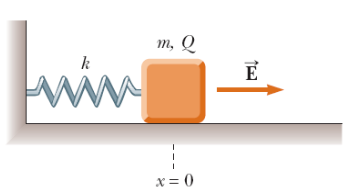(i) If the block is released at rest when the spring is unstretched (at x = 0), by what maximum amount does the spring expand?
(ii) What is the equilibrium position of the block where the acceleration on the block is 0?
(iii) Explain why the block is, or is not, in simple harmonic motion.

Get Help By Expert

Struggling with your PC1201 Fundamental of Physics Assignment at the National University Singapore (NUS)? Our Assignment Writing Service Singapore is here to assist you with TMA, individual assignments, and even Dissertation Writing Services. We understand the challenges you face with velocity graphs and motion problems. Let our experts guide you through. Pay for our assistance and excel in your course confidently.

Categories:-##### No Need To Pay Extra
• Turnitin Report

\$10.00

\$9.00
Per Page
• Consultation with Expert

\$35.00
Per Hour
• Live Session 1-on-1

\$40.00
Per 30 min.
• Quality Check

\$25.00
##### Free

New Special Offer

## Get 30% Off

UP TO 15 % DISCOUNT

Get Your Assignment Completed At Lower Prices

Plagiarism Free Solutions
100% Original Work
24*7 Online Assistance
Native PhD Experts
Hire a Writer Now
My Assignment Help SG Services
My Assignment Help SG